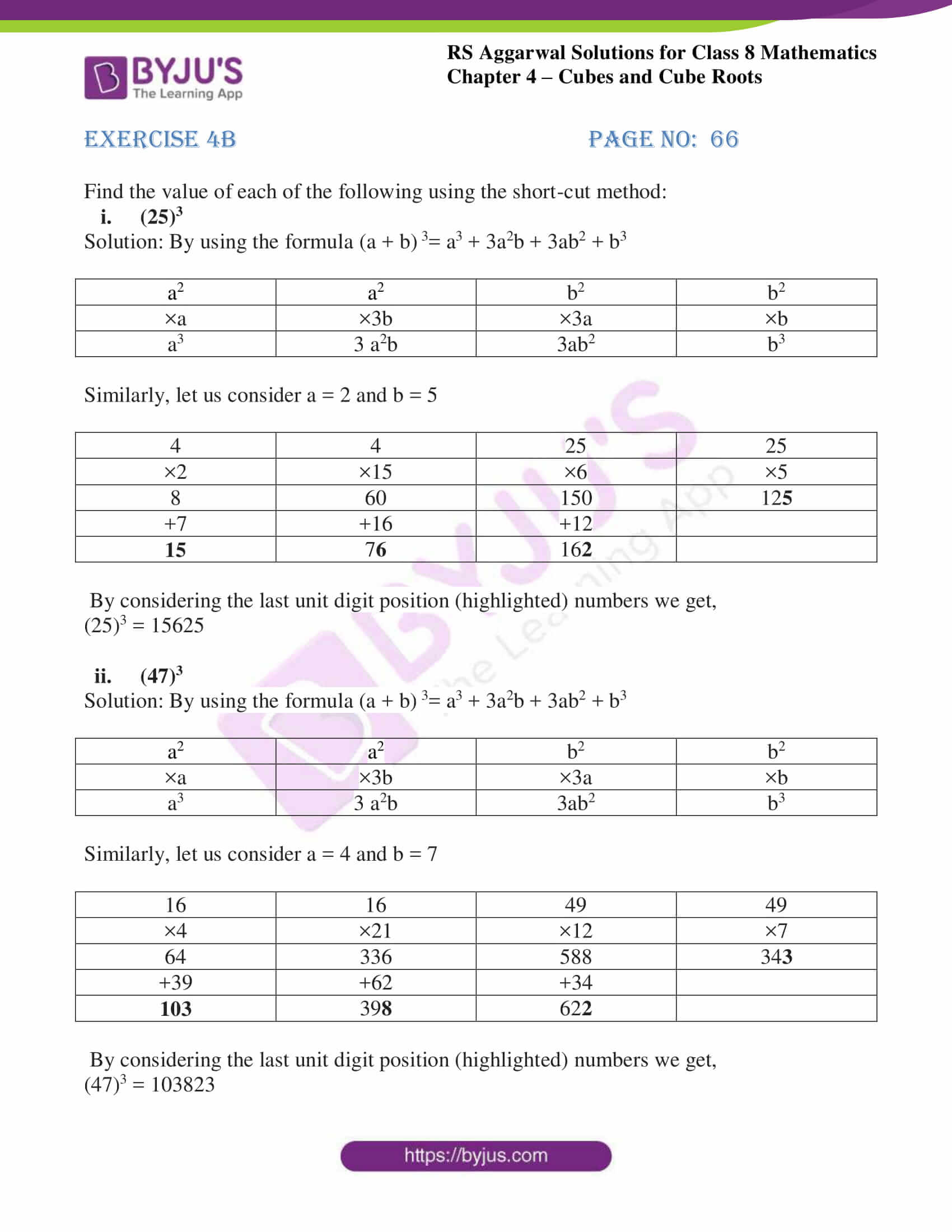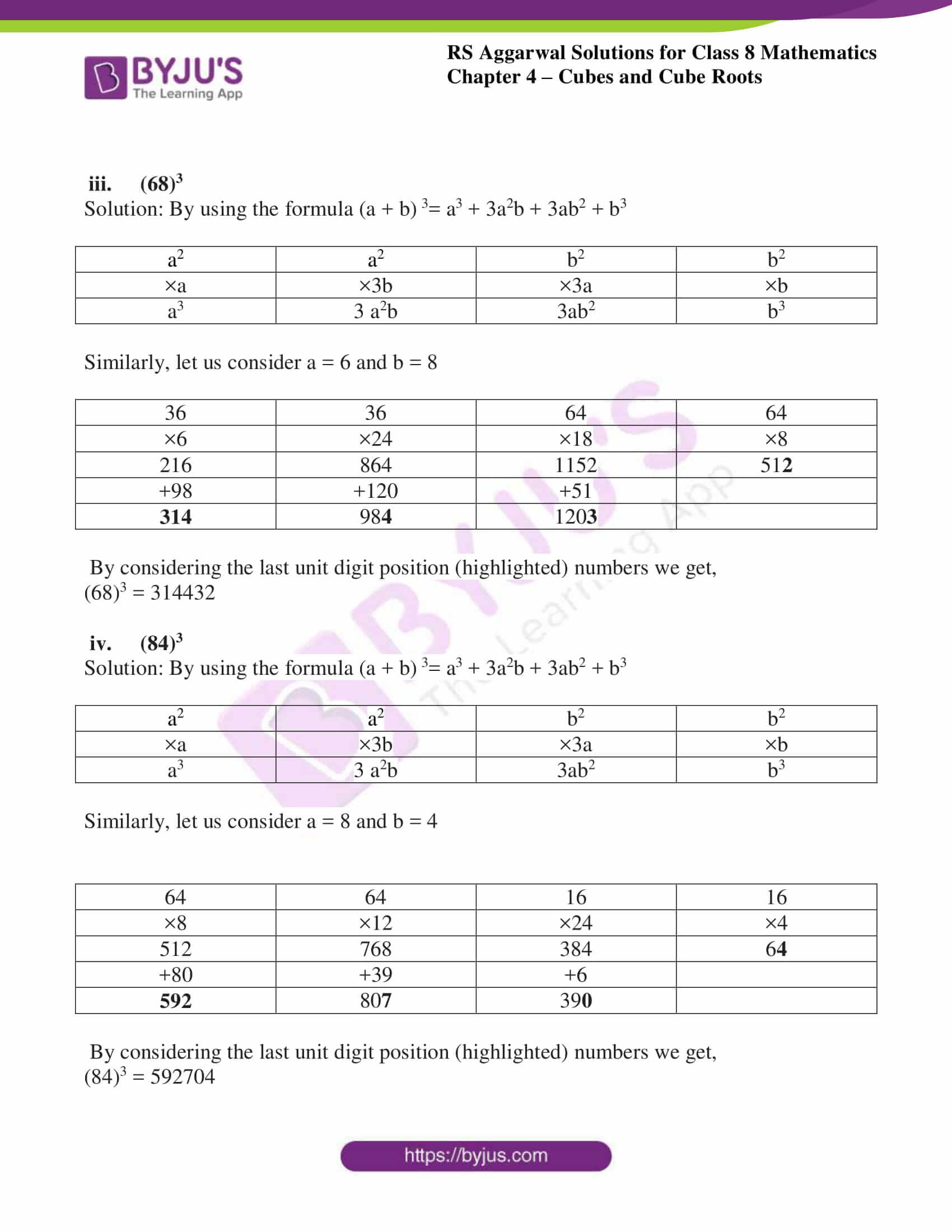# RS Aggarwal Solutions for Class 8 Chapter 4 - Cubes and Cube Roots Exercise 4B

Our expert team has solved the RS Aggarwal Solutions to ensure that the students are thorough with their basic concepts by practicing the solutions. Students can refer and download RS Aggarwal Solutions for Class 8 Maths Chapter 4- Exercise 4B, Cubes and Cube Roots from the links provided below. In Exercise 4B of RS Aggarwal Class 8 Maths, we shall study the methods used to find the cube of a two-digit number.

## Download PDF of RS Aggarwal Solutions for Class 8 Maths Chapter 4- Exercise 4B### Access Answers to RS Aggarwal Solutions for Class 8 Maths Chapter 4- Cubes and Cube Roots Exercise 4B

Find the value of each of the following using the short-cut method:

i. (25)3

Solution: By using the formula (a + b) 3= a3 + 3a2b + 3ab2 + b3

 a2 a2 b2 b2 ×a ×3b ×3a ×b a3 3 a2b 3ab2 b3

Similarly, let us consider a = 2 and b = 5

 4 4 25 25 ×2 ×15 ×6 ×5 8 60 150 125 +7 +16 +12 15 76 162

By considering the last unit digit position (highlighted) numbers we get,

(25)3 = 15625

ii. (47)3

Solution: By using the formula (a + b) 3= a3 + 3a2b + 3ab2 + b3

 a2 a2 b2 b2 ×a ×3b ×3a ×b a3 3 a2b 3ab2 b3

Similarly, let us consider a = 4 and b = 7

 16 16 49 49 ×4 ×21 ×12 ×7 64 336 588 343 +39 +62 +34 103 398 622

By considering the last unit digit position (highlighted) numbers we get,

(47)3 = 103823

iii. (68)3

Solution: By using the formula (a + b) 3= a3 + 3a2b + 3ab2 + b3

 a2 a2 b2 b2 ×a ×3b ×3a ×b a3 3 a2b 3ab2 b3

Similarly, let us consider a = 6 and b = 8

 36 36 64 64 ×6 ×24 ×18 ×8 216 864 1152 512 +98 +120 +51 314 984 1203

By considering the last unit digit position (highlighted) numbers we get,

(68)3 = 314432

iv. (84)3

Solution: By using the formula (a + b) 3= a3 + 3a2b + 3ab2 + b3

 a2 a2 b2 b2 ×a ×3b ×3a ×b a3 3 a2b 3ab2 b3

Similarly, let us consider a = 8 and b = 4

 64 64 16 16 ×8 ×12 ×24 ×4 512 768 384 64 +80 +39 +6 592 807 390

By considering the last unit digit position (highlighted) numbers we get,

(84)3 = 592704

## RS Aggarwal Solutions for Class 8 Maths Chapter 4- Exercise 4B

Exercise 4B of RS Aggarwal Class 8, Cubes and Cube Roots contains advance concepts related to Cubes. This exercise mainly deals with the short-cut method for finding the cube of a two-digit number by using the column method.

The RS Aggarwal Solutions can help the students practice at ease while learning the fundamentals as it provides all the answers to the questions from the RS Aggarwal textbook. Practicing as many times as possible helps in a better understanding of the concepts and also helps in improving time management skills and also boosts the confidence level to achieve high marks.